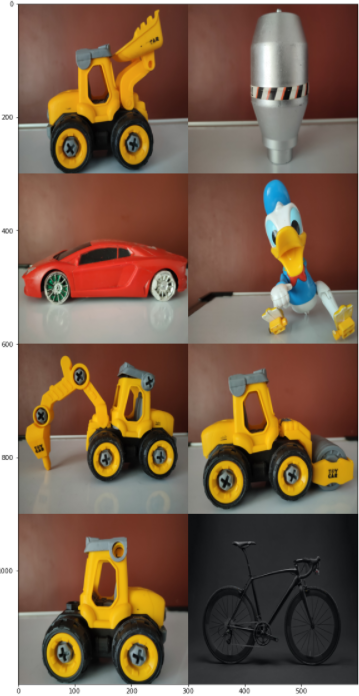# Show images in grid inside jupyter notebook using matplotlib and numpy

Posted on May 11, 2021 · 5 mins read

In this post, we will see how to display the images in a grid using matplotlib imshow and Image grid. Alternatively, we will see the visualization of image blocks and show it in a grid using numpy

This entire exercise is done using a jupyter notebook, so it may be useful for someone who would like to visualize their images in a grid format inside a cell of Jupyter notebook.

These are three ways that will be demonstrated in this post for displaying the images in grid

1. Matplotlib imshow
2. Matplotlib Image Grid
3. Numpy

Let’s get started, first we will define a function to load and resize the images and convert that into a numpy array

``````import matplotlib.pyplot as plt
import numpy as np
import os
from PIL import Image

def img_reshape(img):
img = Image.open('./images/'+img).convert('RGB')
img = img.resize((300,300))
img = np.asarray(img)
return img
``````

## Sample Image

Let’s see a sample image using the above method img_reshape(), before that let’s create the array of all the image data so it will be easier to iterate and choose an image from the list

``````images = os.listdir('./images')
img_arr = []

for image in images:
img_arr.append(img_reshape(image))
``````

Now we have an img_arr that contains all the available image data, Let’s display an image from this list and see how our sample image looks like

``````pil_im = img_reshape('img4.jpg')
plt.imshow(np.asarray(pil_im))
``````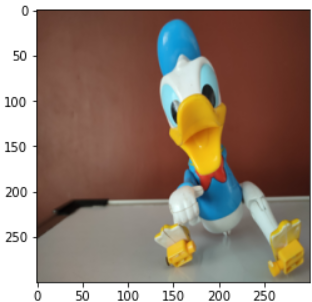## Show mutliple images using matplotlib imshow

Let’s take first four images and display that in a 2x2 grid, first we have to create a subplot with nrows = 2 and ncols = 2 and set a figure size of 15, 15. The variable img_count is used to iterate through the image index in the array(img_arr) created above

``````rows=2
cols = 2
img_count = 0

fig, axes = plt.subplots(nrows=rows, ncols=cols, figsize=(15,15))

for i in range(rows):
for j in range(cols):
if img_count < len(images):
axes[i, j].imshow(img_dict[img_count])
img_count+=1
``````

This will display the first four images in a 2x2 grid as shown in this figure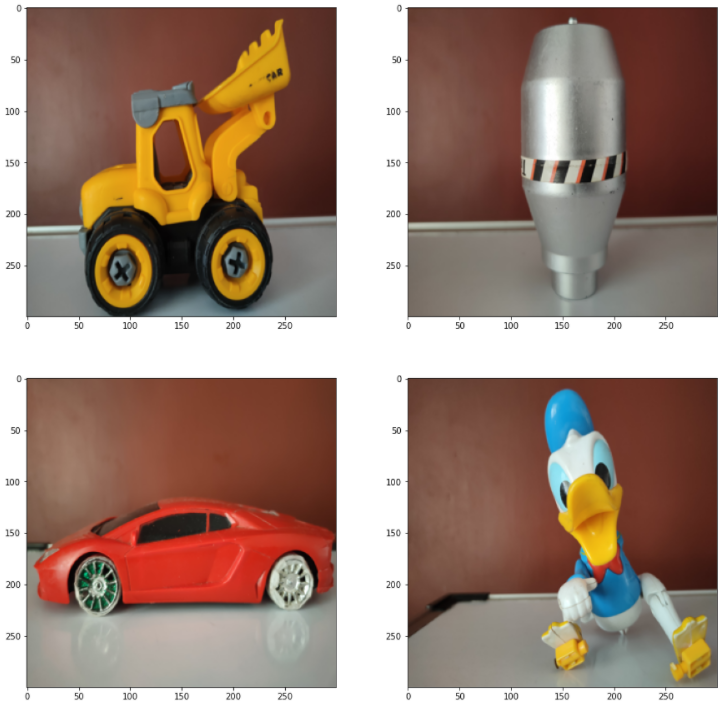## Matplotlib Image grid

It’s a class that creates grids of axes, we can align multiple images of different size using it, The ImageGrid() function here will take the following four arguments:

1. fig - It’s the parent figure for displaying images
2. rect - This will set the axes position as `(left, bottom, width, height)` tuple or as a three-digit subplot position code, it’s 111 in our case
3. nrows_ncols - number of rows and cols, the shape of the grid, it’s 2x2 in our case here
4. axes - Horizontal or Vertical padding between axes in inches
``````import matplotlib.pyplot as plt
from mpl_toolkits.axes_grid1 import ImageGrid
import numpy as np

fig = plt.figure(figsize=(20., 20.))
grid = ImageGrid(fig, 111,
nrows_ncols=(2, 2),  # creates 2x2 grid of axes
)

for ax, im in zip(grid, img_arr):
ax.imshow(im)

plt.show()

``````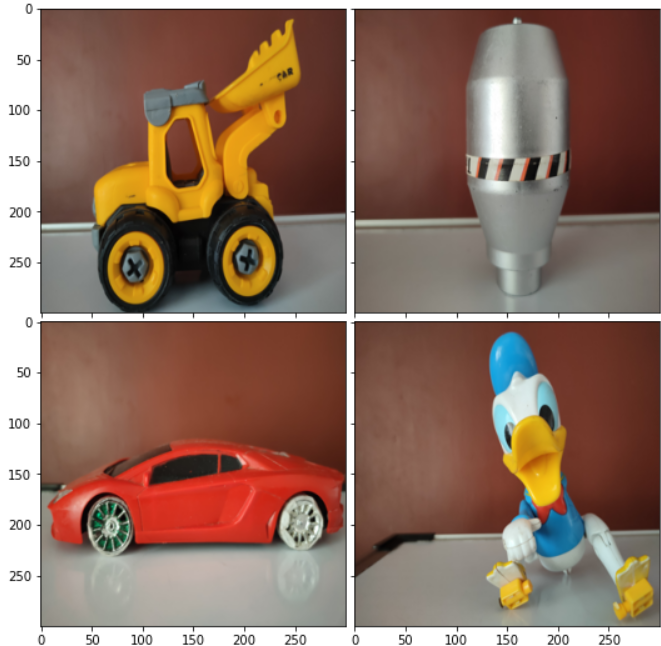## Image grid using numpy

Let’s display all our images in a 2x4 grid using numpy, we will use the image array(img_arr) created above and then we will create a function that takes image array and number of columns as second argument

Inside the function, the image array will be reshaped and split the first axes into two axes, one of length nrows and one of length ncols. Then swapaxes(1,2) to reorder the axes

``````def image_grid(array, ncols=4):
index, height, width, channels = array.shape
nrows = index//ncols

img_grid = (array.reshape(nrows, ncols, height, width, channels)
.swapaxes(1,2)
.reshape(height*nrows, width*ncols, intensity))

return img_grid

result = image_grid(np.array(img_arr))
fig = plt.figure(figsize=(20., 20.))
plt.imshow(result)
``````

Here is the output for a 2x4 and 4x2 grid using numpy

2x4 grid: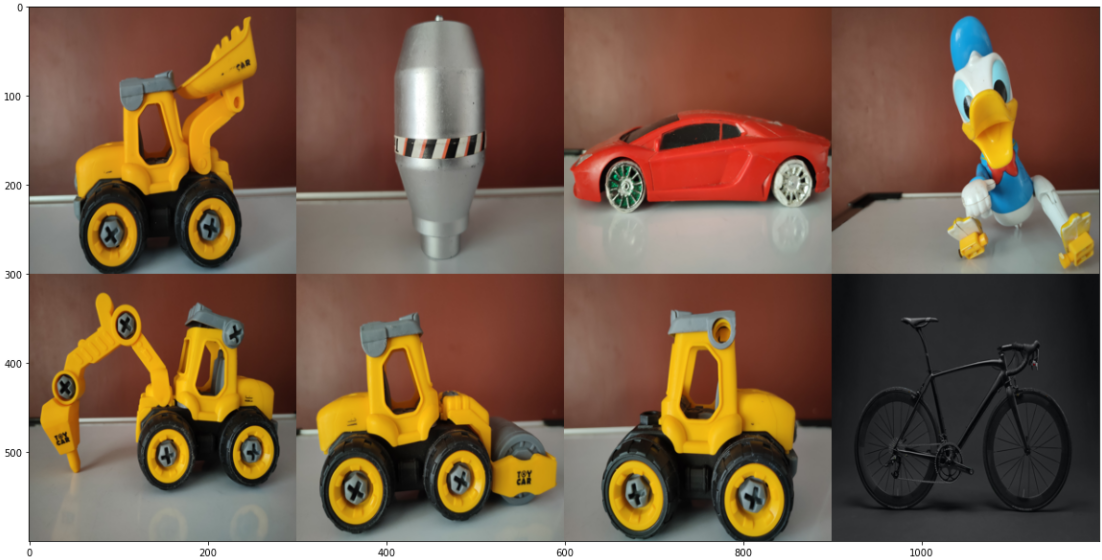4x2 grid: